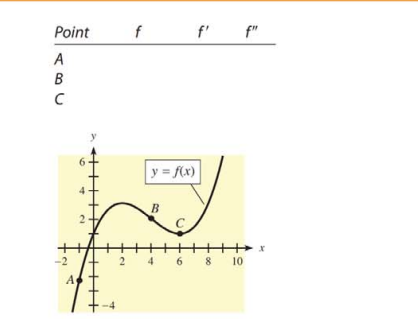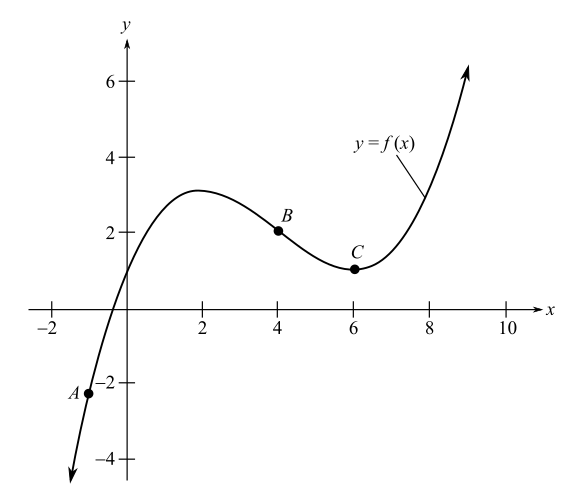Chapter 10, Problem 9T### Mathematical Applications for the ...

11th Edition
Ronald J. Harshbarger + 1 other
ISBN: 9781305108042

#### Solutions

Chapter
Section### Mathematical Applications for the ...

11th Edition
Ronald J. Harshbarger + 1 other
ISBN: 9781305108042
Textbook Problem

# Use the following graph of y = f(x) and the indicated points to complete the chart. Enter +, -, or 0 according to whether f, f', and f" are positive, negative, or zero at each point. Point f f' f'' A B CTo determine

The values in the table, enter +, or 0 according to whether f, f' and f" are positive, negative or zero at each point for the graph of the function.

 Point f f' f" A B CExplanation

Given Information:

The provided graph of the function is

Explanation:

Consider the provided graph of the function,

From the graph at point A, the value of f(x) is negative and at points B and C, the value of f(x) is positive.

Since, at A the function f(x) is increasing on both left and right of A, the value of f'(x) is positive, at point B, f(x) is decreasing on both left and right of B, the value of f'(x) is negative and point C, f(x) is decreasing on the left side and increasing on the right side, the value of f'(x) is 0 at C.

Recall that if f(x)>0 and f(x) is concave up and if f(x)<0 and f(x) is concave down

### Still sussing out bartleby?

Check out a sample textbook solution.

See a sample solution

#### The Solution to Your Study Problems

Bartleby provides explanations to thousands of textbook problems written by our experts, many with advanced degrees!

Get Started